# Selina solutions for Concise Maths Class 10 ICSE chapter 14 - Equation of a Line [Latest edition]

#### Chapters## Chapter 14: Equation of a Line

Exercise 14 (A)Exercise 14 (B)Exercise 14 (C)Exercise 14 (D)Exercise 14 (E)
Exercise 14 (A) [Pages 185 - 186]

### Selina solutions for Concise Maths Class 10 ICSE Chapter 14 Equation of a Line Exercise 14 (A) [Pages 185 - 186]

Exercise 14 (A) | Q 1.1 | Page 185

Find, if (1,3) lie on the line x – 2y + 5 = 0

Exercise 14 (A) | Q 1.2 | Page 185

Find, if point (0,5) lie on the line x – 2y + 5 = 0

Exercise 14 (A) | Q 1.3 | Page 185

Find, if point (-5,0) lie on the line x – 2y + 5 = 0

Exercise 14 (A) | Q 1.4 | Page 185

Find, if point (5,5) lie on the line x – 2y + 5 = 0

Exercise 14 (A) | Q 1.5 | Page 185

Find, if point (2,-1.5) lie on the line x – 2y + 5 = 0

Exercise 14 (A) | Q 1.6 | Page 185

Find, if point (-2,-1.5) lie on the line x – 2y + 5 = 0

Exercise 14 (A) | Q 2.1 | Page 185

State, true or false : the line x/ y+y/3=0 passes through the point (2, 3)

Exercise 14 (A) | Q 2.2 | Page 185

State, true or false: the line x/2+y/3=0 passes through the point (4, -6)

Exercise 14 (A) | Q 2.3 | Page 185

State, true or false: the point (8, 7) lies on the line y – 7 = 0

Exercise 14 (A) | Q 2.4 | Page 185

State, true or false: the point (-3, 0) lies on the line x + 3 = 0

Exercise 14 (A) | Q 2.5

State, true or false: if the point (2, a) lies on the line 2x – y = 3, then a = 5.

Exercise 14 (A) | Q 3 | Page 185

The line given by the equation 2x-y/3=7 passes through the point (k, 6); calculate the value of k.

Exercise 14 (A) | Q 4 | Page 185

For what value of k will the point (3, −k) lie on the line 9x + 4y = 3?

Exercise 14 (A) | Q 5 | Page 185

The line (3x)/5-(2y)/3+1=0 contains the point (m, 2m – 1); calculate the value of m.

Exercise 14 (A) | Q 6 | Page 186

Does the line 3x − 5y = 6 bisect the join of (5, −2) and (−1, 2)?

Exercise 14 (A) | Q 7.1 | Page 186

the line y = 3x – 2 bisects the join of (a, 3) and (2, −5), Find the value of a.

Exercise 14 (A) | Q 7.2 | Page 186

the line x – 6y + 11 = 0 bisects the join of (8, −1) and (0, k). Find the value of k.

Exercise 14 (A) | Q 8.1 | Page 186

the point (−3, 2) lies on the line ax + 3y + 6 = 0, calculate the value of a.

Exercise 14 (A) | Q 8.2 | Page 186

The line y = mx + 8 contains the point (−4, 4), calculate the value of m.

Exercise 14 (A) | Q 9 | Page 186

The point P divides the join of (2, 1) and (−3, 6) in the ratio 2 : 3. Does P lies on the line x − 5y + 15 = 0?

Exercise 14 (A) | Q 10 | Page 186

The line segment joining the points (5, −4) and (2, 2) is divided by the points Q in the ratio 1:2 Does the line x – 2y = 0 contain Q?

Exercise 14 (A) | Q 11 | Page 186

Find the point of intersection of the lines: 4x + 3y = 1 and 3x − y + 9 = 0. If this point lies on the line (2k – 1) x – 2y = 4; find the value of k.

Exercise 14 (A) | Q 12 | Page 186

Show that the lines 2x + 5y = 1, x – 3y = 6 and x + 5y + 2 = 0 are concurrent.

Exercise 14 (B) [Pages 190 - 191]

### Selina solutions for Concise Maths Class 10 ICSE Chapter 14 Equation of a Line Exercise 14 (B) [Pages 190 - 191]

Exercise 14 (B) | Q 1.1 | Page 190

Find the slope of the line whose inclination is: 0°

Exercise 14 (B) | Q 1.2 | Page 190

Find the slope of the line whose inclination is: 30°

Exercise 14 (B) | Q 1.3 | Page 190

Find the slope of the line whose inclination is: 72° 30'

Exercise 14 (B) | Q 1.4 | Page 190

Find the slope of the line whose inclination is: 46°

Exercise 14 (B) | Q 2.1 | Page 190

Find the inclination of the line whose slope is: 0

Exercise 14 (B) | Q 2.2 | Page 190

Find the inclination of the line whose slope is: sqrt3

Exercise 14 (B) | Q 2.3 | Page 190

Find the inclination of the line whose slope is: 0.7646

Exercise 14 (B) | Q 2.4 | Page 190

Find the inclination of the line whose slope is: 1.0875

Exercise 14 (B) | Q 3.1 | Page 190

Find the slope of the line passing through the (−2, −3) and (1, 2)

Exercise 14 (B) | Q 3.2 | Page 190

Find the slope of the line passing through the (−4, 0) and origin

Exercise 14 (B) | Q 3.3 | Page 190

Find the slope of the line passing through the (a, −b) and (b, −a)

Exercise 14 (B) | Q 4.1 | Page 190

Find the slope of the line parallel to AB if: A = (−2, 4) and B = (0, 6)

Exercise 14 (B) | Q 4.2 | Page 190

Find the slope of the line parallel to AB if: A = (0, −3) and B = (−2, 5)

Exercise 14 (B) | Q 5.1 | Page 190

Find the slope of the line perpendicular to AB if: A = (0, −5) and B = (−2, 4)

Exercise 14 (B) | Q 5.2 | Page 190

Find the slope of the line perpendicular to AB if: A = (3, −2) and B = (−1, 2)

Exercise 14 (B) | Q 6 | Page 190

The line passing through (0, 2) and (−3, −1) is parallel to the line passing through (-1, 5) and (4,a), Find a.

Exercise 14 (B) | Q 7 | Page 190

The line passing through (−4, −2) and (2, −3) is perpendicular to the line passing through (a, 5) and (2, −1). Find a.

Exercise 14 (B) | Q 8 | Page 190

Without using the distance formula, show that the points A (4, −2), B (−4, 4) and C (10, 6) are the vertices of a right angled triangle.

Exercise 14 (B) | Q 9 | Page 190

Without using the distance formula, show that the points A (4, 5), B (1, 2), C (4, 3) and D (7, 6) are the vertices of a parallelogram.

Exercise 14 (B) | Q 10 | Page 190

(−2, 4), B (4, 8), C (10, 7) and D (11, -5) are the vertices of a quadrilateral. Show that the quadrilateral, obtained on joining the mid-points of its sides, is a parallelogram.

Exercise 14 (B) | Q 11 | Page 190

Show that the points P (a, b + c), Q (b, c + a) and R (c, a + b) are collinear.

Exercise 14 (B) | Q 12 | Page 190

Find x, if the slope of the line joining (x, 2) and (8, −11) is (−3)/4

Exercise 14 (B) | Q 13 | Page 191

The side AB of an equilateral triangle ABC is parallel to the x-axis. Find the slopes of all its sides.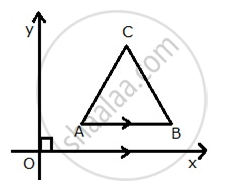Exercise 14 (B) | Q 14 | Page 191

The side AB of a square ABCD is parallel to x-axis. Find the slopes of all its sides. Also, find:
(i) the slope of the diagonal AC.
(ii) the slope of the diagonal BD.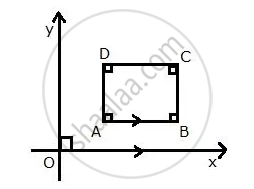Exercise 14 (B) | Q 15 | Page 191

A (5, 4), B (−3, −2) and C (1, −8) are the vertices of a triangle ABC. Find:
(i) the slope of the altitude of AB.
(ii) the slope of the median AD and
(iii) the slope of the line parallel to AC.

Exercise 14 (B) | Q 16 | Page 191

The slope of the side BC of a rectangle ABCD is 2/3
Find:
(i) the slope of the side AB.
(ii) the slope of the side AD.

Exercise 14 (B) | Q 17.1 | Page 191

Find the slope and the inclination of the line AB if :

A = (−3, −2) and B = (1, 2)

Exercise 14 (B) | Q 17.2 | Page 191

Find the slope and the inclination of the line AB if:

A = (0, - sqrt3 ) and B = (3, 0)

Exercise 14 (B) | Q 17.3 | Page 191

Find the slope and the inclination of the line AB if:

A = (−1, 2 sqrt3 ) and B = (−2, sqrt3 )

Exercise 14 (B) | Q 18 | Page 191

The points (−3, 2), (2, −1) and (a, 4) are collinear Find a.

Exercise 14 (B) | Q 19 | Page 191

The points (K, 3), (2, −4) and (-K + 1, −2) are collinear. Find K.

Exercise 14 (B) | Q 20 | Page 191

Plot the points A (1, 1), B (4, 7) and C(4, 10) on a graph paper. Connect A and B and also A and C.
Which segment appears to have the steeper slope, AB or AC?
Justify your conclusion by calculating the slopes of AB and AC.

Exercise 14 (B) | Q 21.1 | Page 191

Find the value(s) of k so that PQ will be parallel to RS. Given: P (2, 4), Q (3, 6), R (8, 1) and S (10, k)

Exercise 14 (B) | Q 21.2 | Page 191

Find the value(s) of k so that PQ will be parallel to RS. Given: P (3, −1), Q (7, 11), R (−1, −1) and S (1, k)

Exercise 14 (B) | Q 21.3 | Page 191

Find the value(s) of k so that PQ will be parallel to RS. Given: P (5, −1), Q (6, 11), R (6, −4k) and S (7, k2)

Exercise 14 (C) [Pages 197 - 198]

### Selina solutions for Concise Maths Class 10 ICSE Chapter 14 Equation of a Line Exercise 14 (C) [Pages 197 - 198]

Exercise 14 (C) | Q 1 | Page 197

Find the equation of a line whose:
y- intercept = 2 and slope = 3

Exercise 14 (C) | Q 2 | Page 197

Find the equation of a line whose:
y – intercept = −1 and inclination = 45°

Exercise 14 (C) | Q 3 | Page 197

Find the equation of the line whose slope is − 4/3 and which passes through (−3, 4)

Exercise 14 (C) | Q 4 | Page 197

Find the equation of a line which passes through (5, 4) and makes an angle of 60° with the positive direction of the x-axis.

Exercise 14 (C) | Q 5.1 | Page 197

Find the equation of the line passing through:  (0, 1) and (1, 2)

Exercise 14 (C) | Q 5.2 | Page 197

Find the equation of the line passing through: (−1, −4) and (3, 0)

Exercise 14 (C) | Q 6.1 | Page 197

The co-ordinates of two points P and Q are (2, 6) and (−3, 5) respectively Find the gradient of PQ;

Exercise 14 (C) | Q 6.2 | Page 197

The co-ordinates of two points P and Q are (2, 6) and (−3, 5) respectively Find the equation of PQ;

Exercise 14 (C) | Q 6.3 | Page 197

The co-ordinates of two points P and Q are (2, 6) and (−3, 5) respectively Find the co-ordinates of the point where PQ intersects the x-axis.

Exercise 14 (C) | Q 7.1 | Page 197

The co-ordinates of two points A and B are (-3, 4) and (2, -1) Find the equation of AB

Exercise 14 (C) | Q 7.2 | Page 197

The co-ordinates of two points A and B are (-3, 4) and (2, -1) Find: the co-ordinates of the point where the line AB intersects the y-axis.

Exercise 14 (C) | Q 8 | Page 197

The figure given alongside shows two straight lines AB and CD intersecting each other at point P (3, 4). Find the equations of AB and CD.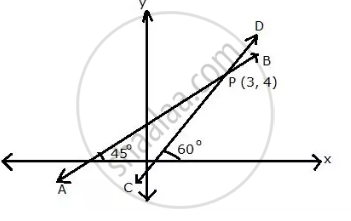Exercise 14 (C) | Q 9 | Page 197

In ΔABC, A(3, 5), B(7, 8) and C(1, –10). Find the equation of the median through A.

Exercise 14 (C) | Q 10 | Page 197

The following figure shows a parallelogram ABCD whose side AB is parallel to the x-axis. ∠A = 60° and vertex C = (7, 5). Find the equations of BC and CD.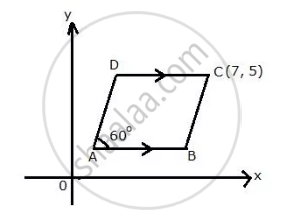Exercise 14 (C) | Q 11 | Page 197

Find the equation of the straight line passing through origin and the point of intersection of the lines x + 2y = 7 and x – y = 4.

Exercise 14 (C) | Q 12 | Page 197

In triangle ABC, the co-ordinates of vertices A, B and C are (4, 7), (-2, 3) and (0, 1) respectively.
Find the equation of median through vertex A.
Also, find the equation of the line through vertex B and parallel to AC.

Exercise 14 (C) | Q 13 | Page 197

A, B and C have co-ordinates (0, 3), (4, 4) and (8, 0) respectively. Find the equation of the line through A and perpendicular to BC.

Exercise 14 (C) | Q 14 | Page 197

Find the equation of the perpendicular dropped from the point (−1, 2) onto the line joining the points (1, 4) and (2, 3)

Exercise 14 (C) | Q 15.1 | Page 197

Find the equation of the line, whose x-intercept = 5 and y-intercept = 3

Exercise 14 (C) | Q 15.2 | Page 197

Find the equation of the line, whose x-intercept = -4 and y-intercept = 6

Exercise 14 (C) | Q 15.3 | Page 197

Find the equation of the line, whose  x-intercept = −8 and y-intercept = -4

Exercise 14 (C) | Q 16 | Page 197

Find the equation of the line whose slope is -5/6 and x-intercept is 6.

Exercise 14 (C) | Q 17 | Page 197

Find the equation of the line with x-intercept 5 and a point on it (-3, 2)

Exercise 14 (C) | Q 18 | Page 197

Find the equations of the line through (1, 3) and making an intercept of 5 on the y-axis.

Exercise 14 (C) | Q 19 | Page 197

Find the equations of the lines passing through point (-2, 0) and equally inclined to the coordinate axes.

Exercise 14 (C) | Q 20.1 | Page 197

The line through P (5, 3) intersects y-axis at Q.
write the slope of the line

Exercise 14 (C) | Q 20.2 | Page 197

The line through P (5, 3) intersects y-axis at Q.
write the equation of the line

Exercise 14 (C) | Q 20.3 | Page 197

The line through P (5, 3) intersects y-axis at Q.

Find the co-ordinates of Q.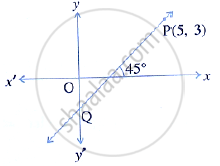Exercise 14 (C) | Q 21 | Page 198

Write down the equation of the line whose gradient is -2/5 and which passes through point P, where P divides the line segement joining A(4, −8) and B (12, 0) in the ratio 3 : 1

Exercise 14 (C) | Q 22.1 | Page 198

A (1, 4), B (3, 2) and C (7, 5) are vertices of a triangle ABC. Find the co-ordinates of the centroid of triangle ABC

Exercise 14 (C) | Q 22.2 | Page 198

A (1, 4), B (3, 2) and C (7, 5) are vertices of a triangle ABC. Find the equation of a line, through the centroid and parallel to AB.

Exercise 14 (C) | Q 23 | Page 198

A (7, −1), B (4, 1) and C (−3, 4) are the vertices of a triangle ABC. Find the equation of a line through the vertex B and the point P in AC; such that AP : CP = 2 : 3.

Exercise 14 (D) [Pages 201 - 202]

### Selina solutions for Concise Maths Class 10 ICSE Chapter 14 Equation of a Line Exercise 14 (D) [Pages 201 - 202]

Exercise 14 (D) | Q 1.1 | Page 201

Find the slope and y-intercept of the line: y = 4

Exercise 14 (D) | Q 1.2 | Page 201

Find the slope and y-intercept of the line: ax – by = 0

Exercise 14 (D) | Q 1.3 | Page 201

Find the slope and y-intercept of the line: 3x – 4y = 5

Exercise 14 (D) | Q 2 | Page 201

The equation of a line is x – y = 4. Find its slope and y – intercept. Also, find its inclination.

Exercise 14 (D) | Q 3.1 | Page 201

Is the line 3x + 4y + 7 = 0 perpendicular to the line 28x – 21y + 50 = 0?

Exercise 14 (D) | Q 3.2 | Page 201

Is the line x – 3y = 4 perpendicular to the line 3x – y = 7?

Exercise 14 (D) | Q 3.3 | Page 201

Is the line 3x + 2y = 5 parallel to the line x + 2y = 1?

Exercise 14 (D) | Q 3.4 | Page 201

Determine x so that slope of the line through (1, 4) and (x, 2) is 2.

Exercise 14 (D) | Q 4.1 | Page 201

Find the slope of the line which is parallel to:

x + 2y + 3 = 0

Exercise 14 (D) | Q 4.2 | Page 201

Find the slope of the line which is parallel to:

x/2 - y/3 -1 = 0

Exercise 14 (D) | Q 5.1 | Page 201

Find the slope of the line which is perpendicular to:

x-y/2 +3=0

Exercise 14 (D) | Q 5.2 | Page 201

Find the slope of the line which is perpendicular to:

x/3 -2y = 4

Exercise 14 (D) | Q 6.1 | Page 201

Lines 2x – by + 5 = 0 and ax + 3y = 2 are parallel to each other. Find the relation connecting a and b.

Exercise 14 (D) | Q 6.2 | Page 201

Lines mx + 3y + 7 = 0 and 5x – ny – 3 = 0 are perpendicular to each other. Find the relation
connecting m and n.

Exercise 14 (D) | Q 7 | Page 201

Find the value of p if the lines, whose equations are 2x – y + 5 = 0 and px + 3y = 4 are
perpendicular to each other.

Exercise 14 (D) | Q 8 | Page 201

The equation of a line AB is 2x – 2y + 3 = 0
(i) Find the slope of line AB.
(ii) Calculate the angle that the line AB makes with the positive direction of the x-axis.

Exercise 14 (D) | Q 9 | Page 201

The lines represented by 4x + 3y = 9 and px - 6y+ 3 = 0 are parallel. Find the value of p.

Exercise 14 (D) | Q 10 | Page 201

If the lines y = 3x + 7 and 2y + px = 3 are perpendicular to each other, find the value of p.

Exercise 14 (D) | Q 11 | Page 201

The line through A (−2, 3) and B (4, b) is perpendicular to the line 2x – 4y = 5. Find the value
of b.

Exercise 14 (D) | Q 12.1 | Page 201

Find the equation of the line passing through (−5, 7) and parallel to: x-axis ?

Exercise 14 (D) | Q 12.2 | Page 201

Find the equation of the line passing through (−5, 7) and parallel to y - axis ?

Exercise 14 (D) | Q 13.1 | Page 201

Find the equation of the line passing through (5, -3) and parallel to x – 3y = 4.

Exercise 14 (D) | Q 13.2 | Page 201

Find the equation of the line parallel to the line 3x + 2y = 8 and passing through the point (0, 1).

Exercise 14 (D) | Q 14 | Page 201

Find the equation of the line passing through (−2, 1) and perpendicular to 4x + 5y = 6.

Exercise 14 (D) | Q 15 | Page 201

Find the equation of the perpendicular bisector of the line segment obtained on joining the points
(6, −3) and (0, 3)

Exercise 14 (D) | Q 16 | Page 201

In the following diagram, write down:
(i) the co-ordinates of the points A, B and C.
(ii) the equation of the line through A and parallel to BC.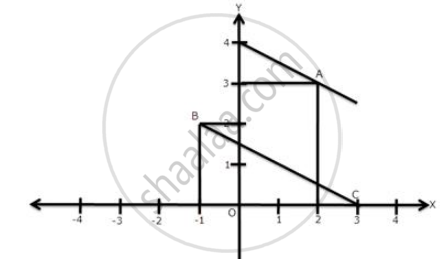Exercise 14 (D) | Q 17 | Page 201

B (−5, 6) and D (1, 4) are the vertices of rhombus ABCD. Find the equations of diagonals BD
and AC.

Exercise 14 (D) | Q 18 | Page 201

A (7, −2) and C = (−1, −6) are the vertices of square ABCD. Find the equations of diagonals AC and BD.

Exercise 14 (D) | Q 19.1 | Page 201

A (1, −5), B (2, 2) and C (−2, 4) are the vertices of triangle ABC, Find the equation of :

the median of the triangle through A.

Exercise 14 (D) | Q 19.2 | Page 201

A (1, −5), B (2, 2) and C (−2, 4) are the vertices of triangle ABC, Find the equation of :
the altitude of the triangle through B.

Exercise 14 (D) | Q 19.3 | Page 201

A (1, −5), B (2, 2) and C (−2, 4) are the vertices of triangle ABC, Find the equation of :

the line through C and parallel to AB.

Exercise 14 (D) | Q 20 | Page 201

(i) Write down the equation of the line AB, through (3, 2) and perpendicular to the line
2y = 3x + 5

(ii) AB meets the x-axis at A and the y-axis at B. Write down the co-ordinates of A and B.
Calculate the area of triangle OAB, where O is the origin.

Exercise 14 (D) | Q 21 | Page 201

The line 4x − 3y + 12 = 0 meets x-axis at A.Write the co-ordinates of A. determine the equation of the line through A and perpendicular to 4x – 3y + 12 = 0

Exercise 14 (D) | Q 22 | Page 201

The point P is the foot of perpendicular from A (−5, 7) to the line whose equation is 2x – 3y + 18 = 0. Determine :
(i) the equation of the line AP
(ii) the co-ordinates of P.

Exercise 14 (D) | Q 23 | Page 202

The points A, B and C are (4, 0), (2, 2) and (0, 6) respectively. Find the equations of AB and
BC. If AB cuts the y-axis at P and BC cuts the x-axis at Q, Find the co-ordinates of P and Q.

Exercise 14 (D) | Q 24 | Page 202

Match the equations A, B, C and D with the lines L1, L2, L3 and L4, whose graphs are roughly drawn in the given diagram.
A ≡ y = 2x ;

B ≡ Y – 2x + 2 = 0

C ≡ 3x + 2y = 6

D ≡ Y = 2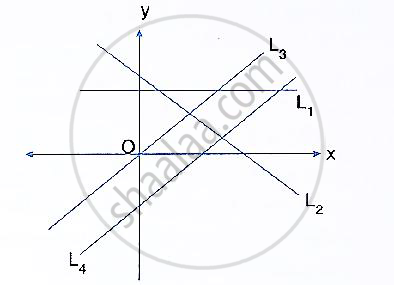Exercise 14 (D) | Q 25 | Page 202

Find the value of a for which the points A(a, 3), B(2, 1) and C(5, a) are collinear. Hence, find the equation of the line.

Exercise 14 (E) [Pages 202 - 204]

### Selina solutions for Concise Maths Class 10 ICSE Chapter 14 Equation of a Line Exercise 14 (E) [Pages 202 - 204]

Exercise 14 (E) | Q 1 | Page 202

Point P divides the line segment joining the points A (8, 0) and B (16, -8) in the ratio 3:5 Find its co-ordinates of point P. Also, find the equation of the line through P and parallel to 3x + 5y = 7

Exercise 14 (E) | Q 2 | Page 202

The line segment joining the points A (3, -4) and B (-2, 1) is divided in the ratio 1 : 3 at point P
in it. Find the co-ordinates of P. Also, find the equation of the line through P and perpendicular to the line 5x – 3y = 4.

Exercise 14 (E) | Q 3 | Page 202

A line 5x + 3y + 15 = 0 meets y – axis at point P. Find the co-ordinates of points P. Find the equation of a line through P and perpendicular to x – 3y + 4 = 0.

Exercise 14 (E) | Q 4 | Page 202

Find the value of K for which the lines kx – 5y + 4 = 0 and 5x – 2y + 5 = 0 are perpendicular to
each other.

Exercise 14 (E) | Q 5 | Page 202

A straight line passes through the points P (-1, 4) and Q (5, -2). It intersects the co-ordinate axes at points A and B. M is the mid-point of the segment AB . Find: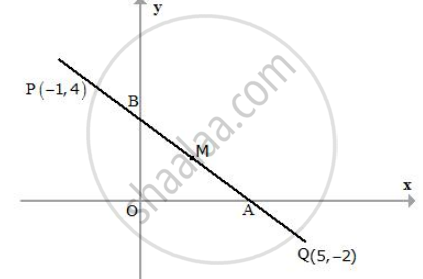(i) The equation of the line
(ii) The co-ordinates of A and B.
(iii) The co-ordinates of M.

Exercise 14 (E) | Q 6 | Page 202

(1, 5) and (-3, -1) are the co-ordinates of vertices A and C respectively of rhombus ABCD. Find
the equations of the diagonals AC and BD.

Exercise 14 (E) | Q 7 | Page 202

Show that A(3, 2), B (6, −2) and C (2, −5) can be the vertices of a square.
(i) Find the co-ordinates of its fourth vertex D, if ABCD is a square
(ii) Without using the co-ordinates of vertex D, find the equation of side AD of the square and
also the equation of diagonal BD.

Exercise 14 (E) | Q 8 | Page 202

A line through origin meets the line x = 3y + 2 at right angles at point X. Find the co-ordinates of X.

Exercise 14 (E) | Q 9 | Page 202

A straight line passes through the point (3, 2) and the portion of this line, intercepted between the positive axes, is bisected at this point. Find the equation of the line.

Exercise 14 (E) | Q 10 | Page 202

Find the equation of the line passing through the point of intersection of 7x + 6y = 71 and 5x –8y = − 23; and perpendicular to the line 4x – 2y = 1

Exercise 14 (E) | Q 11 | Page 202

Find the equation of the line which is perpendicular to the line x/a - y/b=1 at the point where this line meets y-axis.

Exercise 14 (E) | Q 12.1 | Page 202

O (0, 0), A (3, 5) and B (−5, −3) are the vertices of triangle OAB. Find the equation of median of triangle OAB through vertex O

Exercise 14 (E) | Q 12.2 | Page 202

O (0, 0), A (3, 5) and B (−5, −3) are the vertices of triangle OAB. Find the equation of altitude of triangle OAB through vertex B.

Exercise 14 (E) | Q 13 | Page 202

Determine whether the line through points (-2, 3) and (4, 1) is perpendicular to the line
3x = y + 1.
Does line 3x = y + 1 bisect the line segment joining the two given points?

Exercise 14 (E) | Q 14 | Page 203

Given a straight line x cos 30° + y sin 30° = 2. Determine the equation of the other line which
is parallel to it and passes through (4, 3).

Exercise 14 (E) | Q 15 | Page 203

Find the value of k such that the line (k - 2)x + (k + 3)y - 5 = 0 is:
(i) perpendicular to the line 2x – y + 7 = 0
(ii) parallel to it.

Exercise 14 (E) | Q 16 | Page 203

The vertices of a triangle ABC are A (0, 5), B (−1, −2) and C (11, 7). Write down the equation of BC Find :
(i)the equation of line through A and perpendicular to BC.

(ii) the co-ordinates of the point P, where the perpendicular through A, as obtained in (i), meets
BC.

Exercise 14 (E) | Q 17 | Page 203

From the given figure, find:
(i) the co-ordinates of A, B and C.
(ii) the equation of the line through A and parallel to BC.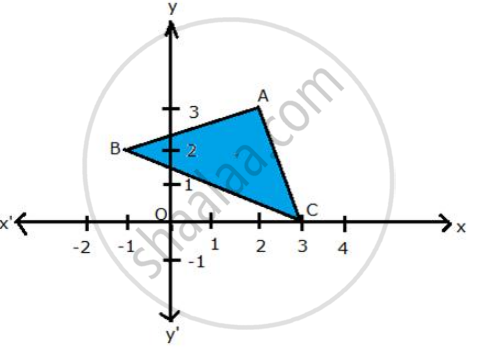Exercise 14 (E) | Q 18 | Page 203

P(3, 4), Q(7, -2) and R(-2, -1) are the vertices of triangle PQR. Write down the equation of the median of the triangle through R.

Exercise 14 (E) | Q 19 | Page 203

A(8, −6), B(−4, 2) and C(0, −10) are vertices of a triangle ABC. If P is the mid-point of AB and Q is the mid-point of AC, use co-ordinate geometry to show that PQ is parallel to BC. Give a special name to quadrilateral PBCQ.

Exercise 14 (E) | Q 20 | Page 203

A line AB meets the x-axis at point A and y-axis at point B. The point P(−4, −2) divides the line segment AB internally such that AP : PB = 1 : 2, Find:
(i) the co-ordinates of A and B
(ii) equation of line through P and perpendicular to AB.

Exercise 14 (E) | Q 21 | Page 203

A line intersects x-axis at point (−2, 0) and cuts off an intercept of 3 units from the positive side of y-axis. Find the equation of the line.

Exercise 14 (E) | Q 22 | Page 203

Find the equation of a line passing through the point (2, 3) and having the x-intercept of 4 units.

Exercise 14 (E) | Q 23 | Page 203

The given figure (not drawn to scale) shows two straight lines AB and CD. If equation of the
line AB is :
Y = x + 1 and equation of line CD is:
y = sqrt3  x – 1. Write down the inclination of lines AB and CD; also, find the angle θ between AB
and CD.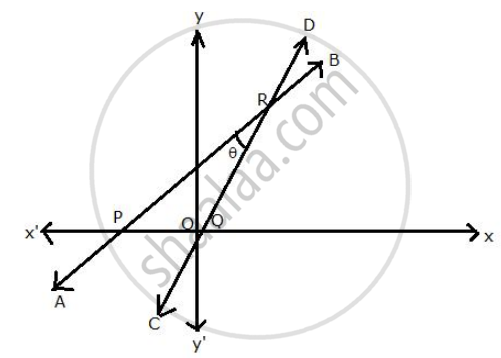Exercise 14 (E) | Q 24 | Page 203

Write down the equation of the line whose gradient is3/2 and which passes through P. where P divides the line segment joining A(−2, 6) and B(3, −4) in the ratio 2 : 3

Exercise 14 (E) | Q 25 | Page 203

The ordinate of a point lying on the line joining the points (6, 4) and (7, -5) is -23. Find the coordinates
of that point.

Exercise 14 (E) | Q 26 | Page 203

Point A and B have Co-ordinates (7, −3) and (1, 9) respectively. Find:
(i) the slope of AB
(ii) the equation of perpendicular bisector of the line segment AB.
(iii) the value of ‘p’ of (-2, p) lies on it.

Exercise 14 (E) | Q 27 | Page 203

A and B are two points on the x-axis and y-axis respectively. P (2, −3) is the mid point of AB Find the
(i) co-ordinates of A and B
(ii) slope of line AB

(iii) equation of line AB

Exercise 14 (E) | Q 28 | Page 203

The equation of a line is 3x + 4y - 7 = 0. Find:
(i) the slope of the line
(ii) the equation of a line perpendicular to the given line and passing through the intersection of
the lines x – y + 2 = 0 and 3x + y – 10 = 0

Exercise 14 (E) | Q 29 | Page 203

ABCD is a parallelogram where A(x, y), B (5, 8), C (4, 7) and D (2, -4). Find :
(i) co-ordinates of A.
(ii) equation of diagonal BD

Exercise 14 (E) | Q 30 | Page 203

Given equation of line L1 is y = 4.
(i) Write the slope of line L2 if L2 is the bisector of angle O.
(ii) write the co-ordinates of point P.
(iii) Find the equation of L2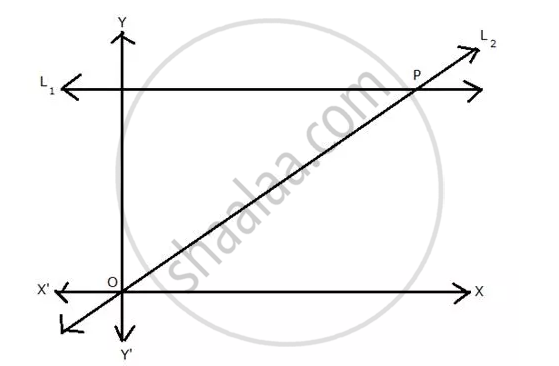Exercise 14 (E) | Q 31.1 | Page 204

Find equation of AB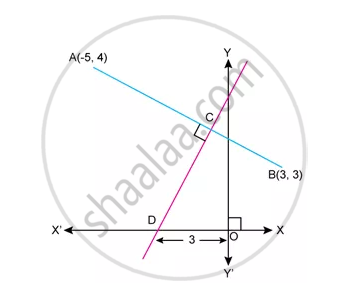Exercise 14 (E) | Q 31.2 | Page 204

Find Equation of CD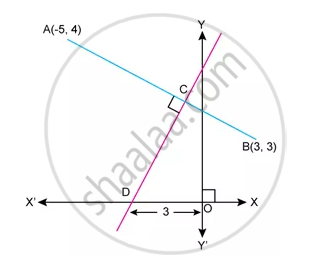Exercise 14 (E) | Q 32 | Page 204

Find the quation of the line that has x-intercept=-3 and -3 and is perpendicular to 3x+5y=1

Exercise 14 (E) | Q 33 | Page 204

A straight line passes the points p(-1,4) and Q(5,-2). It intersects x-axis at point A and y-axis at point B.M is mid-t point of the line segment AB.find:

(1) the equation of the line.

(2) the co-ordinates of point A and B

(3) the co-ordinates of point M

Exercise 14 (E) | Q 34 | Page 204

In the given figure. line AB meets y-axis at point A. Line through C(2,10) and D intersects line AB at right angle at poiunt R find

(1) equation of line AB

(2) equation of line CD

(3) Co-ordinates of point E and D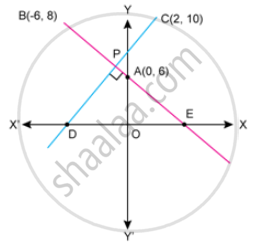Exercise 14 (E) | Q 35 | Page 204

A line through point P(4,3) meets x-axis at point A and the y-axis at point B. If BP is double of PA, find the equation of AB.

Exercise 14 (E) | Q 36 | Page 204

find the equation of line through the line 2x-y=1 and 3x+2y=-9 and making an angle of 30° with positive direction of x-axis.

Exercise 14 (E) | Q 37 | Page 204

Find the equation of the line through the points A(-1,3) and B(0,2). Hence, show that the pointA,B and C (1,1) are collinear.

Exercise 14 (E) | Q 38 | Page 204

Three vertices of a parallelogram ABCD taken in order are A(3,6),B(5,10) and C(3,2), find :

(1) the co-ordinates of the fourth vertex D.

(2) Length of diagonal BD.

(3) equation of side AB of the parallelogram ABCD

Exercise 14 (E) | Q 39 | Page 204

In the figure, ABC is a triangle and BC is parallel to the y-axis. AB and AC intersect the y-axis at P and Q respectively.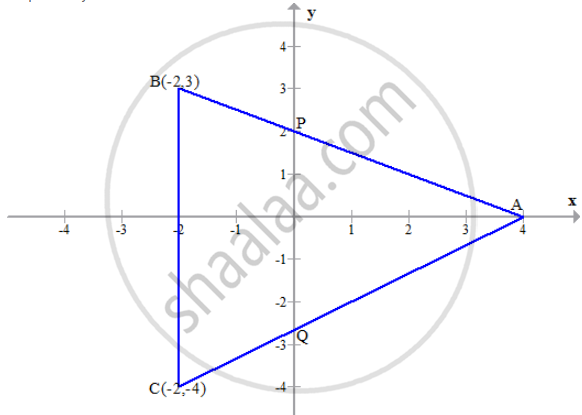(1) Write the co-ordinates of A.

(2) Find the length of Ab and AC.

(3) Find the radio in which Q divides Ac.

(4)find the equation of the line AC.

Exercise 14 (E) | Q 40 | Page 204

The slope of aline joining P(6,k) and Q(1 - 3k, 3) is 1/2 Find
(i) k.
(ii) mid-point of PQ, using the value of 'k' found in (i).

Exercise 14 (E) | Q 41 | Page 204

A line AB meets X-axis at A and Y-axis at B. P(4, -1) divides AB in the ration 1 : 2.

1. Find the co-ordinates of A and B.
2. Find the equation of the line through P and perpendicular to AB.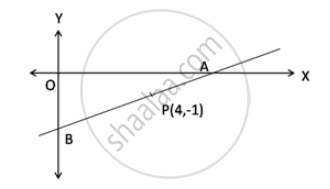## Chapter 14: Equation of a Line

Exercise 14 (A)Exercise 14 (B)Exercise 14 (C)Exercise 14 (D)Exercise 14 (E)## Selina solutions for Concise Maths Class 10 ICSE chapter 14 - Equation of a Line

Selina solutions for Concise Maths Class 10 ICSE chapter 14 (Equation of a Line) include all questions with solution and detail explanation. This will clear students doubts about any question and improve application skills while preparing for board exams. The detailed, step-by-step solutions will help you understand the concepts better and clear your confusions, if any. Shaalaa.com has the CISCE Concise Maths Class 10 ICSE solutions in a manner that help students grasp basic concepts better and faster.

Further, we at Shaalaa.com provide such solutions so that students can prepare for written exams. Selina textbook solutions can be a core help for self-study and acts as a perfect self-help guidance for students.

Concepts covered in Concise Maths Class 10 ICSE chapter 14 Equation of a Line are Slope of a Line, Concept of Slope, Equation of a Line, Various Forms of Straight Lines, General Equation of a Line, Slope – Intercept Form, Two - Point Form, Geometric Understanding of ‘m’ as Slope Or Gradient Or tanθ Where θ Is the Angle the Line Makes with the Positive Direction of the x Axis, Geometric Understanding of c as the y-intercept Or the Ordinate of the Point Where the Line Intercepts the y Axis Or the Point on the Line Where x=0, Conditions for Two Lines to Be Parallel Or Perpendicular, Simple Applications of All Co-ordinate Geometry..

Using Selina Class 10 solutions Equation of a Line exercise by students are an easy way to prepare for the exams, as they involve solutions arranged chapter-wise also page wise. The questions involved in Selina Solutions are important questions that can be asked in the final exam. Maximum students of CISCE Class 10 prefer Selina Textbook Solutions to score more in exam.

Get the free view of chapter 14 Equation of a Line Class 10 extra questions for Concise Maths Class 10 ICSE and can use Shaalaa.com to keep it handy for your exam preparation